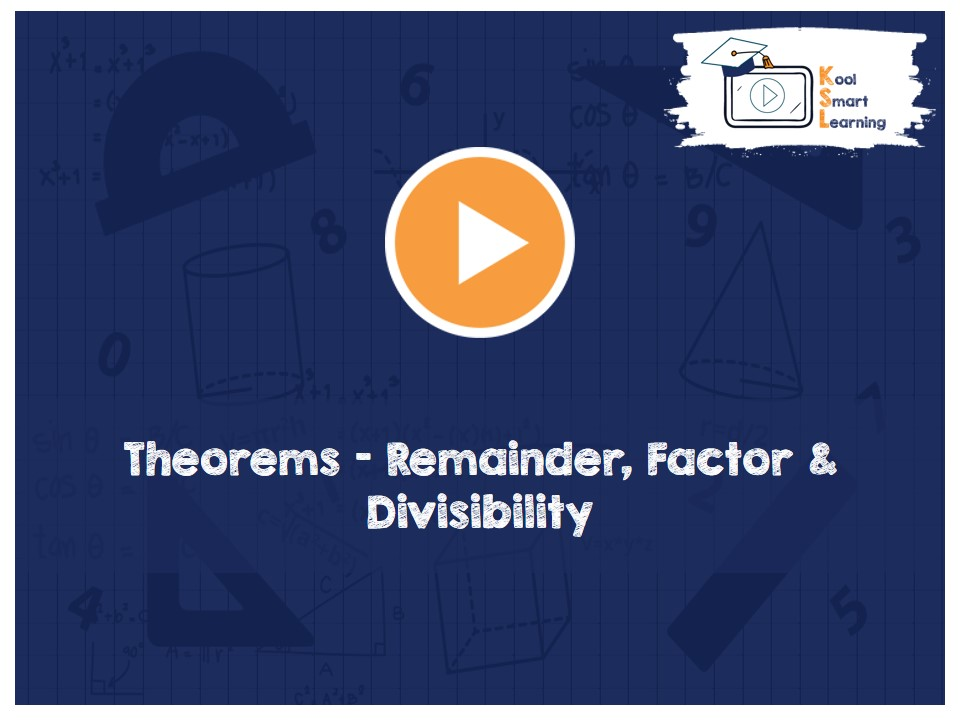At KoolSmartLearning, we intend to harness the power of online education to make learning easy.## Theorems – Remainder, Factor & Divisibility

/  Theorems – Remainder, Factor & Divisibility## Theorems – Remainder, Factor & Divisibility

This video covers the different theorems namely Remainder Theorem, Factor Theorem & Divisibility theorems for expressions like (aˆn+bˆn) and (aˆn-bˆn) divisibility with (a+b) & (a-b) . These theorems are very useful in polynomials. This video also includes questions on the usage of these theorems.

More Related Videos